Multiprocess
Multilevel Modeling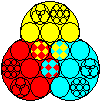aML

product info
multilevel example
multiprocess example

## A Multilevel Example with Unobserved Heterogeneity

Consider a simple multilevel linear regression model given by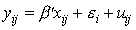where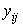is a continuous outcome of observation i, replication j. The equation consists of regressors,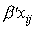, a residual (heterogeneity) that is common to all replications,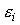, and an iid residual,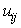. In aML, you may specify this model as follows:

```define regressor set BetaX;
var = <list of variables X>;

define normal distribution; dim=1; name=eps;
define normal distribution; dim=1; name=u;

continuous model;
outcome = y;
model = regressor set BetaX +
residual(draw=1, ref=eps) +
residual(draw=_iid, ref=u);
```
Without going into details, note the following aspects. We defined a "regressor set" BetaX, which corresponds to, and two univariate distributions with residuals eps and u, corresponding toand, respectively. These definitions correspond to estimable model parameters: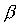and two standard deviations,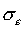and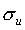. The regressor set and residuals were used to specify the model for outcome y (). The residuals were specified with "draws," which is aML's approach to indicating which residuals are correlated with other residuals in potentially large systems of equations. We specified residual eps with draw=1, i.e., the same draw (the same realization) applies to all repeated outcomes. By contrast, we indicated that residual u is drawn independently (draw=_iid) for every outcome.

How does aML know that there are repeated measures of outcome y, i.e., that this is a multilevel model? This is a data issue. When we created the data (not shown here), outcome variable y was among variables at a lower level of aggregation. During the estimation stage, the level of a variable is of no concern; aML will automatically include as many likelihood modules as there are outcome measures. Explanatory variablesmay be at the same level asor at more aggregated levels.

The example generalizes to all other types of outcomes that aML support, such as durations (hazard models), categorical outcomes (simple/ordered/multinomial probit and logit models), and count outcomes (Poisson, binomial, and negative binomial models). Outcome types may also be mixed, as illustrated in the next example.

To multiprocess example